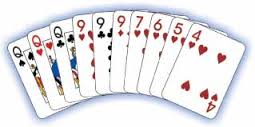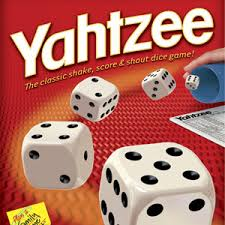• K-5 AIS Math

with Mrs. Smith

• What is AIS math?

AIS math is a remedial service that is provided to students who are below grade level. My services are provided outside of the regular classroom and, depending on the students' needs, are provided for 30 minutes either every other day or on a daily basis. My groups are organzied by both grade and skill level and consist of only one, two, or three students.The small group dynamics allow me to provide intensive services that consist of research based strategies and activities that are aligned to the Common Core Standards and are geared towards meeting each student's individual needs.

• Classroom Rules

1.  Respect each other, our classroom, and out materials

2.  Follow directions the first time they are given

• What Does My Child Need To Know?

Here is a list of many, but not all, of the skills
will be learning this year.  For a complete
list of the Common Core State Standards

Kindergarten

- Counting to 100 by ones and tens

- Identifying and writing numbers 0 to 20

- Comparing two numbers between 0 and 10

- Counting objects using one to one correspondence

-Identifying 2D shapes and describing their attributes
(ex. number of sides, corners)

-Finding the missing number in a sequence of numbers

-Identifying 1 more/1 less

-Counting backwards from 10

-Adding and subtracting using pictures and manipulatives

- Solving basic addition facts using preferred strategies
(ex. doubles, doubles +1, ten facts)

-Solveing basic subtraction facts using a variety of strategies
(ex. number line, counting backwards, counting on)

-Relating addition to subtraction and vice versa
(ex. 3 + 2 = 5, 5 - 3 = 2, 5 - 2 = 3)

- Understanding place value and value up to the tens place

- Skip counting

-Comparing 2-digit numbers

(ex. 1 + __ = 6)

-Telling time to the whole hour

-Using math vocabulary words
(ex. addend, sum, digit, difference, fact families)

- Building fluency with the addition facts up to 10+10

- Adding/subtracting up to 3-digit numbers with regrouping

-Identifying numbers as even or odd

-Understanding place value up to the hundreds place
(ex. expanded, written, standard forms)

-Building fluency with the basic subtraction facts

-Comparing up to 3-digit numbers using >, < = symbols

-Adding/subtracting 10 mentally from a 2-digit number

-Adding/subtracting 100 mentally from a 3-digit number

- Rounding up to 4-digit numbers to any place value

- Understanding place value up to the ten thousands place
(ex. expanded, standard written form)

- Adding/subtracting multi-digit numbers with regrouping

-Understanding what multiplication is

- Memorizing the basic multiplication facts up to 10 x 10

- Place value up to 1,000,000
(ex. expanded, standard, written form)

- Rounding multi-digit numbers to any place value

-Finding factors and multiples

-Solving multi-step word problems

-Multiplying up to 4-digit numbers by a 1-digit number

-Multiplying 2-digit by 2-digit numbers

-Dividing up to a 4-digit dividend by a 1-digit divisor

- Multiplying up to 4-digit numbers by 3-digit numbers
(including both whole numbers and decimals)

- Solving expressions using the Order of Operations

-Identifying prime/composite numbers

-Prime factorization

-Place value
(whole numbers up to billions, decimals to thousandths)

-Rounding whole numbers and decimals

Division with up to a 4-digit dividend and 2-digit divisor
(including both whole numbers and decimals)

-Long Division with interpreting remainders

-Solving multi-step word problems

• Family Game Night

Games not only enhance math skills, but are a great way to spend time with your loved ones.  Pick one set night during the week to play games with your family...and HAVE FUN!!

Here are some classic games that use math skills:

Yahtzee, Monopoly (non-electronic version), Go Fish, Cribbage, Skip Bo (card game), Phase 10 (card game), and any games that require you to keep score (Rummy, Scrabble)• DON'T FORGET...

You can practice your math skills online as well. Check out all of the websites under the "Useful Links" tab.

•MATH-A-THON

Math-A-Thon, America's largest education-based fundraiser, benefits St. Jude Children’s Research Hospital.  To participate, kids ask family members and friends for pledges to solve math problems in the St. Jude Math-A-Thon Funbook, developed by Scholastic.

Hartford has proudly participated in this program
for over 25 years!